Anúncio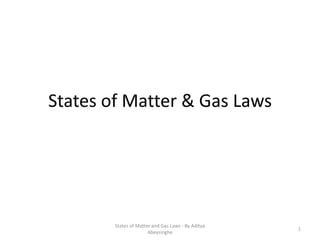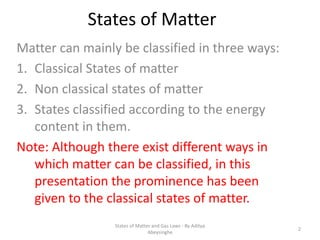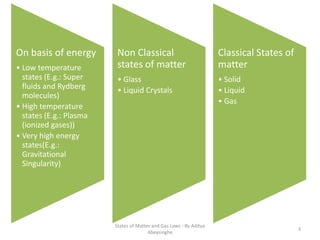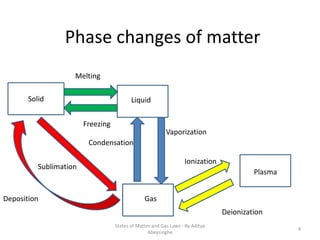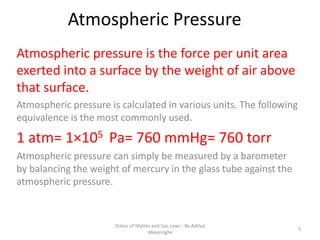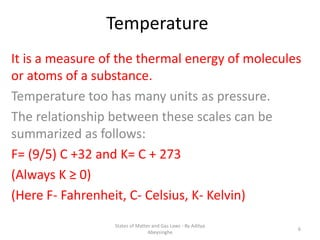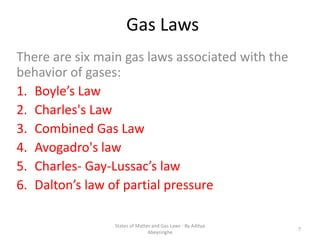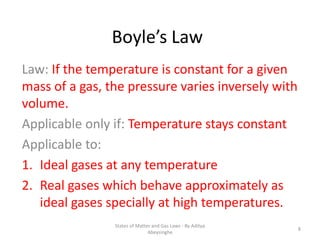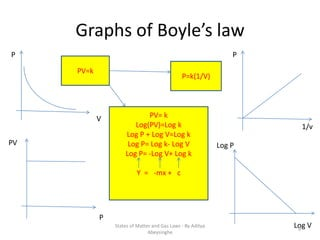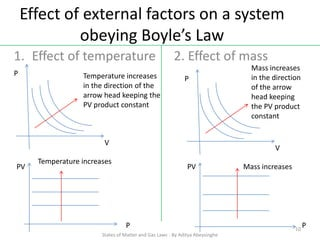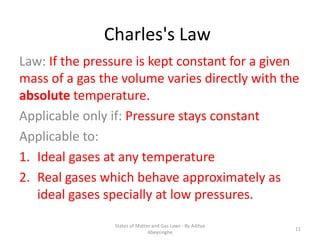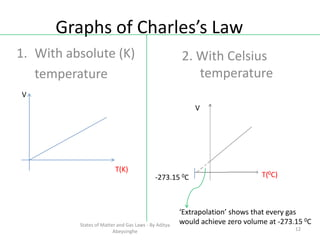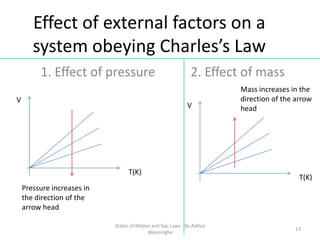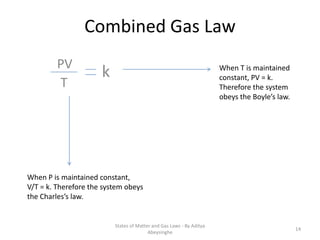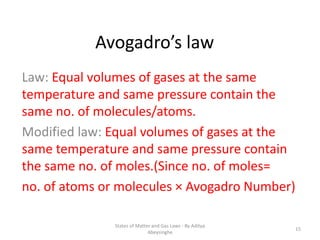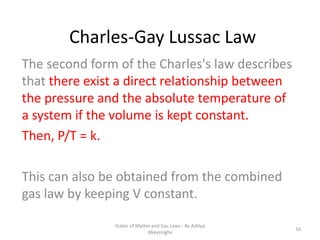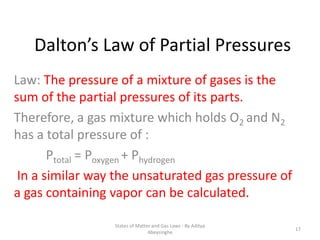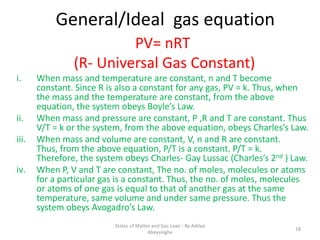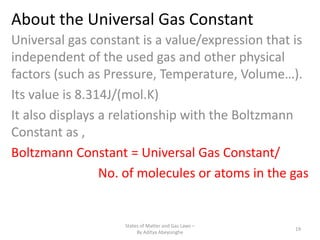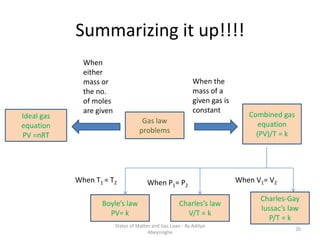1 de 20
Anúncio

### States of matter & gas laws

1. States of Matter & Gas Laws States of Matter and Gas Laws - By Aditya Abeysinghe 1
2. States of Matter Matter can mainly be classified in three ways: 1. Classical States of matter 2. Non classical states of matter 3. States classified according to the energy content in them. Note: Although there exist different ways in which matter can be classified, in this presentation the prominence has been given to the classical states of matter. States of Matter and Gas Laws - By Aditya Abeysinghe 2
3. On basis of energy • Low temperature states (E.g.: Super fluids and Rydberg molecules) • High temperature states (E.g.: Plasma (ionized gases)) • Very high energy states(E.g.: Gravitational Singularity) Non Classical states of matter • Glass • Liquid Crystals Classical States of matter • Solid • Liquid • Gas States of Matter and Gas Laws - By Aditya Abeysinghe 3
4. Phase changes of matter Solid Liquid Gas Plasma Melting Freezing Deposition Sublimation Vaporization Condensation Deionization Ionization States of Matter and Gas Laws - By Aditya Abeysinghe 4
5. Atmospheric Pressure Atmospheric pressure is the force per unit area exerted into a surface by the weight of air above that surface. Atmospheric pressure is calculated in various units. The following equivalence is the most commonly used. 1 atm= 1×105 Pa= 760 mmHg= 760 torr Atmospheric pressure can simply be measured by a barometer by balancing the weight of mercury in the glass tube against the atmospheric pressure. States of Matter and Gas Laws - By Aditya Abeysinghe 5
6. Temperature It is a measure of the thermal energy of molecules or atoms of a substance. Temperature too has many units as pressure. The relationship between these scales can be summarized as follows: F= (9/5) C +32 and K= C + 273 (Always K ≥ 0) (Here F- Fahrenheit, C- Celsius, K- Kelvin) States of Matter and Gas Laws - By Aditya Abeysinghe 6
7. Gas Laws There are six main gas laws associated with the behavior of gases: 1. Boyle’s Law 2. Charles's Law 3. Combined Gas Law 4. Avogadro's law 5. Charles- Gay-Lussac’s law 6. Dalton’s law of partial pressure States of Matter and Gas Laws - By Aditya Abeysinghe 7
8. Boyle’s Law Law: If the temperature is constant for a given mass of a gas, the pressure varies inversely with volume. Applicable only if: Temperature stays constant Applicable to: 1. Ideal gases at any temperature 2. Real gases which behave approximately as ideal gases specially at high temperatures. States of Matter and Gas Laws - By Aditya Abeysinghe 8
9. Graphs of Boyle’s law P V PV=k P=k(1/V) P 1/v PV P PV= k Log(PV)=Log k Log P + Log V=Log k Log P= Log k- Log V Log P= -Log V+ Log k Y = -mx + c Log P Log VStates of Matter and Gas Laws - By Aditya Abeysinghe 9
10. Effect of external factors on a system obeying Boyle’s Law 1. Effect of temperature 2. Effect of mass Temperature increases in the direction of the arrow head keeping the PV product constant P V P V Mass increases in the direction of the arrow head keeping the PV product constant Temperature increases Mass increasesPV PV P P States of Matter and Gas Laws - By Aditya Abeysinghe 10
11. Charles's Law Law: If the pressure is kept constant for a given mass of a gas the volume varies directly with the absolute temperature. Applicable only if: Pressure stays constant Applicable to: 1. Ideal gases at any temperature 2. Real gases which behave approximately as ideal gases specially at low pressures. States of Matter and Gas Laws - By Aditya Abeysinghe 11
12. Graphs of Charles’s Law 1. With absolute (K) temperature V T(K) 2. With Celsius temperature ‘Extrapolation’ shows that every gas would achieve zero volume at -273.15 0C -273.15 0C V T(0C) States of Matter and Gas Laws - By Aditya Abeysinghe 12
13. Effect of external factors on a system obeying Charles’s Law 1. Effect of pressure 2. Effect of mass V T(K) V T(K) Pressure increases in the direction of the arrow head Mass increases in the direction of the arrow head States of Matter and Gas Laws - By Aditya Abeysinghe 13
14. Combined Gas Law PV T k When P is maintained constant, V/T = k. Therefore the system obeys the Charles’s law. When T is maintained constant, PV = k. Therefore the system obeys the Boyle’s law. States of Matter and Gas Laws - By Aditya Abeysinghe 14
15. Avogadro’s law Law: Equal volumes of gases at the same temperature and same pressure contain the same no. of molecules/atoms. Modified law: Equal volumes of gases at the same temperature and same pressure contain the same no. of moles.(Since no. of moles= no. of atoms or molecules × Avogadro Number) States of Matter and Gas Laws - By Aditya Abeysinghe 15
16. Charles-Gay Lussac Law The second form of the Charles's law describes that there exist a direct relationship between the pressure and the absolute temperature of a system if the volume is kept constant. Then, P/T = k. This can also be obtained from the combined gas law by keeping V constant. States of Matter and Gas Laws - By Aditya Abeysinghe 16
17. Dalton’s Law of Partial Pressures Law: The pressure of a mixture of gases is the sum of the partial pressures of its parts. Therefore, a gas mixture which holds O2 and N2 has a total pressure of : Ptotal = Poxygen + Phydrogen In a similar way the unsaturated gas pressure of a gas containing vapor can be calculated. States of Matter and Gas Laws - By Aditya Abeysinghe 17
18. General/Ideal gas equation PV= nRT (R- Universal Gas Constant) i. When mass and temperature are constant, n and T become constant. Since R is also a constant for any gas, PV = k. Thus, when the mass and the temperature are constant, from the above equation, the system obeys Boyle’s Law. ii. When mass and pressure are constant, P ,R and T are constant. Thus V/T = k or the system, from the above equation, obeys Charles’s Law. iii. When mass and volume are constant, V, n and R are constant. Thus, from the above equation, P/T is a constant. P/T = k. Therefore, the system obeys Charles- Gay Lussac (Charles’s 2nd ) Law. iv. When P, V and T are constant, The no. of moles, molecules or atoms for a particular gas is a constant. Thus, the no. of moles, molecules or atoms of one gas is equal to that of another gas at the same temperature, same volume and under same pressure. Thus the system obeys Avogadro’s Law. States of Matter and Gas Laws - By Aditya Abeysinghe 18
19. About the Universal Gas Constant Universal gas constant is a value/expression that is independent of the used gas and other physical factors (such as Pressure, Temperature, Volume…). Its value is 8.314J/(mol.K) It also displays a relationship with the Boltzmann Constant as , Boltzmann Constant = Universal Gas Constant/ No. of molecules or atoms in the gas States of Matter and Gas Laws – By Aditya Abeysinghe 19
20. Summarizing it up!!!! Ideal gas equation PV =nRT Gas law problems Combined gas equation (PV)/T = k Charles-Gay lussac’s law P/T = k Charles’s law V/T = k Boyle’s law PV= k When either mass or the no. of moles are given When the mass of a given gas is constant When T1 = T2 When P1= P2 When V1= V2 States of Matter and Gas Laws - By Aditya Abeysinghe 20
Anúncio